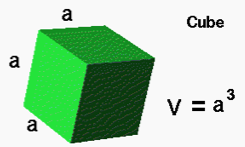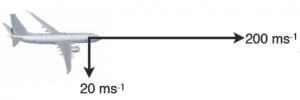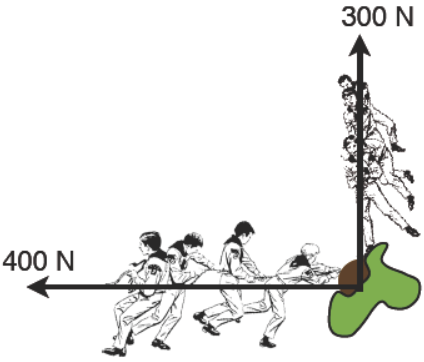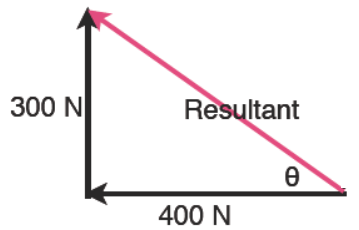Volume is a scalar, it has size but no direction

A vector is a measurement that has both size and direction. For example a force has a size measured in newtons and will be acting up, down, sideways or in some other direction direction.

Velocity is another vector you need to be practiced with. Velocity is given in metres per second squared and a direction east, west, towards the north or some other direction.

Other examples of vectors include weight (a force which as far as we are concerned always acts "downwards"), acceleration and electric current.

A scalar is a quantity with size but no direction, for example speed (we talk about the top speed of a car but don't say which way it has to go) mass, volume and area.Velocity is a vector having size and direction. Speed however only has size, so it is a scalar

Example  An aircraft is flying due east but there is a strong north wind blowing. Which way is the aircraft heading and how fast?

First add the vectors tip to tip. (in black, scale 40 m/s = 1 cm) The resultant, in red, is drawn from the start to the finish.  If you draw this to scale then you can find the resultant by measuring the length and converting back to the velocity. Similarly you can measure angle θ with a protractor.Example 2

For example two teams of boy scouts work together to pull down a tree. They are not very bright so they don’t pull in the same direction, they pull at 90 degrees to one another.

We can find the total force by representing each this as a vector diagram where the length of the line represents the force. If we choose a simple

scale of one cm = 100N and then add the vectors tip to tip. It does not matter which we start with:We can find the exact value of the resultant (the combination of the two) by using an accurate scale diagram or by a calculation.

Because we have a right angle we can find the resultant using Pythagoras:

RxR = 300x300 + 400x400

R is therefore the square root of 25,000

so R = 500

To find the angle we use one of the trig functions, for example: sinθ = (opposite) 300/ (hypotenuse) 500    so sinθ =0.6

giving θ as 36.9 degrees

You can see and download easily printable PDF of notes here:  Adding vectors     Resolving vectors

Or for a short video lesson, watch below.     To go back to the resources index page click here:  https://www.fizzics.org/fizzics-guide/